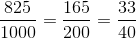## Example Questions

### Example Question #2 : Decimals

Write 0.45 as a fraction.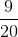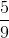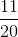Explanation:

.45 is equivalent to 45 out of 100, or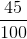.

Divide both the numerator and denominator by 5 to simplify the fraction:### Example Question #2 : Decimals With Fractions

A tub of food containspounds of vegetables,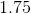pounds of lard, andpounds of sausage.  What is its total weight as an improper fraction?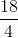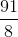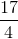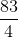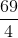Explanation:

To begin with, it is easiest just to add these decimals together using your calculator: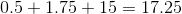Now, this is the same thing as: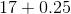We can rewrite this: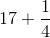To find this, you need to give the two numbers a common denominator: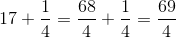### Example Question #2 : How To Find The Fractional Equivalent Of A Decimal

What is the fractional equivalent of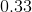?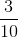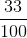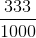Explanation:

In decimal formis said 33 hundredths.

This is equal to.

This fraction cannot be reduced any further therefore it is in its final answer form.

### Example Question #1 : How To Find The Fractional Equivalent Of A Decimal

Convert the decimal to fraction form and reduce it to its simplest form.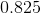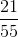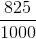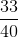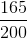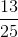In order to convertto a fraction, you would first begin with, because the decimal literally reads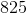thousandths. You can reduce bya few times or just begin by dividing both numbers byto get.